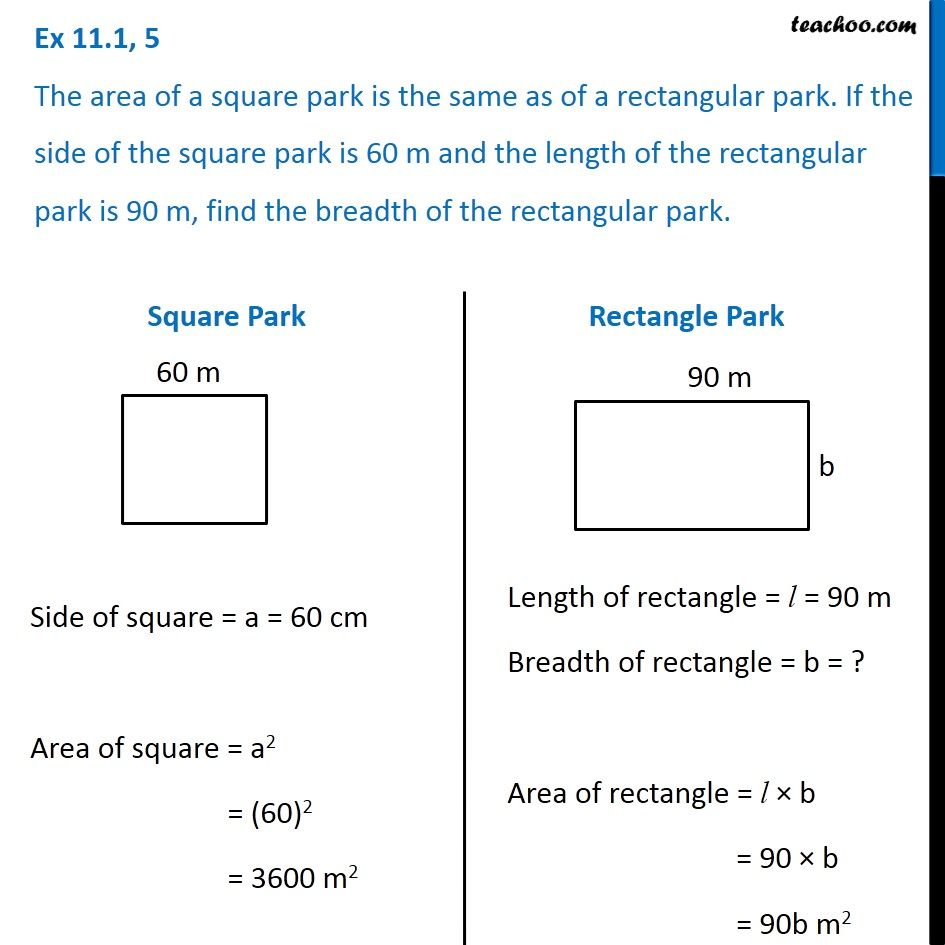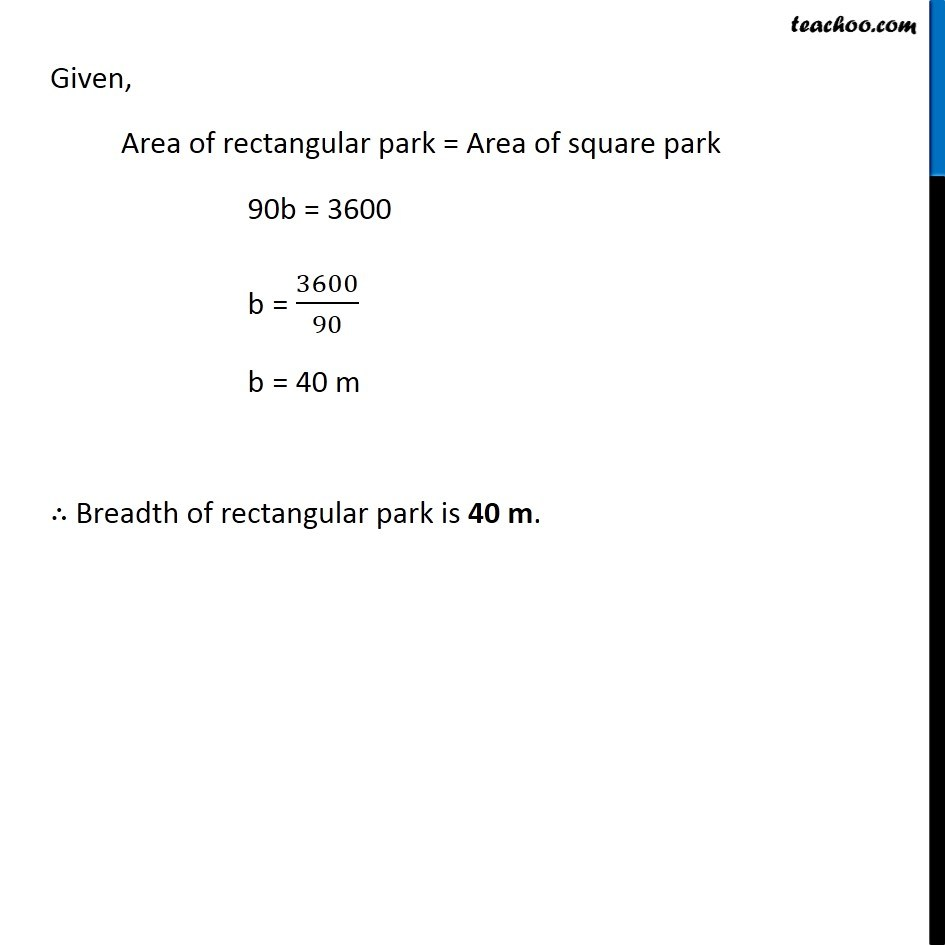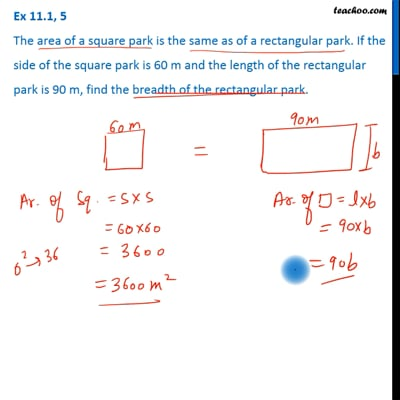Ex 11.1

Chapter 11 Class 7 Perimeter and Area
Serial order wiseThis video is only available for Teachoo black users

Get live Maths 1-on-1 Classs - Class 6 to 12

### Transcript

Ex 11.1, 5 The area of a square park is the same as of a rectangular park. If the side of the square park is 60 m and the length of the rectangular park is 90 m, find the breadth of the rectangular park. Square Park Side of square = a = 60 cm Area of square = a2 = (60)2 = 3600 m2 Rectangle Park Length of rectangle = l = 90 m Breadth of rectangle = b = ? Area of rectangle = l × b = 90 × b = 90b m2 Given, Area of rectangular park = Area of square park 90b = 3600 b = 3600/90 b = 40 m ∴ Breadth of rectangular park is 40 m.